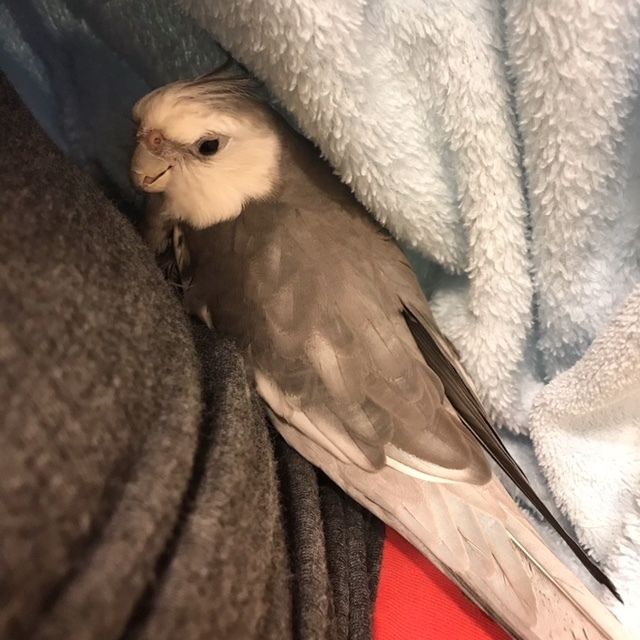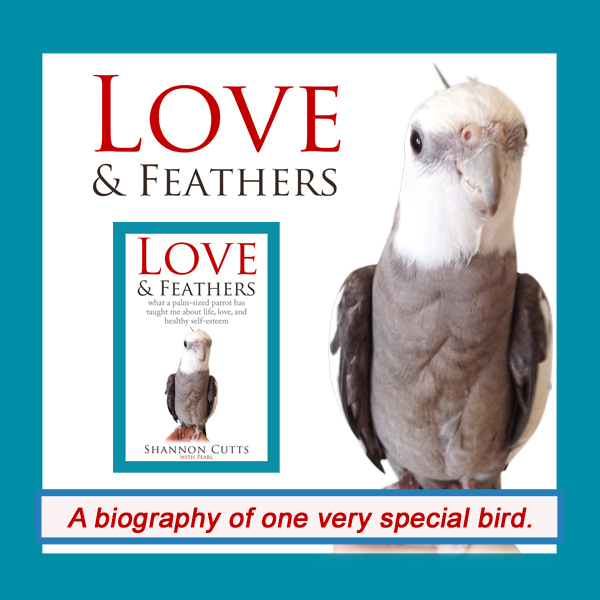# November 2018

 table div table+table+table div table{width:100%;padding:0}table div table+table+table div table img{width:96.23%;padding:0;float:none}table div table+table+table div table td{width:100%;padding:0 1.88% 18px}/* styles *//* styles */ Receiving this email as a forward? Sign up to keep following our little flock's adventures!
 table div table+table+table+table+table+table div table{width:100%;padding:0}table div table+table+table+table+table+table div table img{width:96.23%;padding:0;float:none}table div table+table+table+table+table+table div table td{width:100%;padding:0 1.88% 18px}/* styles */## Where to Go When You Don't Know Where to GoWhen it is cold outside, the very best place to be is next to your mommy, nestled inside your warm blue fuzzy bathrobe!

"Where to go when you don't know where to go."

At first glance, this sounds like one of those frustrating statements that initially sounds profound but ends up meaning nothing.

But when the phrase popped into my mind during my yoga practice this morning, something clicked.

Part of it was timing. Sometimes during yoga practice, my mind becomes calm - like when my online teacher, Adriene, specifically says "now, give your thinking mind a break."

But at other times, like this morning, my mind doesn't think it needs a break. It has so much to think about! Often what it is thinking about upsets me and feels a lot like a critique of how well (or not) I'm living my life to date.

So when, all of a sudden, right in the middle of a long mental monologue about how my life is going nowhere and may have actually passed me up a long time ago, I heard "Where to go when you don't know where to go?"

Like one of those incomprehensible riddles meditation teachers give their students, this stopped my mind cold. "Hmmmm," it thought. "Where to go when I don't know where to go?"

And it started thinking about that instead. Eventually, miraculously, it concluded the right place to go is always within, deep, deep within, not stopping until all feels completely quiet, silent, still.

Stillness, it decided, is the "within place" where actual guidance about next steps, or simply waiting with enhanced patience reserves, is available and free for the asking. Inside that within place of stillness, I can find calm, reassurance, friendship, compassion, encouragement, even a chin up "atta girl" if I need one.

In that "within place" is pure stillness, but there is also everything I love most - nature, the ocean, the trees, wind, sunshine, rain, the breath, the cheerful sound of my parrot chirping, the sight of my two precious shells placidly surveying their turf, my dearest loved ones (human and not human), meditation, yoga, color, light, rest, peace - all of it.

When I go there, to that within place, the comparisons and competitiveness and feeling of having wasted every opportunity I've ever received and missed the boat so many times that boats themselves are now obsolete, it all dissipates. It dissolves into a sea of wisdom that says I am not the only being who has ever felt this way or had these worries and survived them.

Then it tells me once again that the life I am seeking is not in these things, these milestones or even the stepping stones to get to the milestones. Where I am going - truly going - none of that matters or exists.

With love, a spirit of service, small kindnesses, humility, the inner smile, the outer smile, laughter, every tiny flutter of love, it all evens out. There is equality, somehow, in the place beyond differences only the outer eye can see and the outer ear can hear.

I am slowly training myself - reminding myself - that there is always a place where I can go when I don't know where to go or what to do or who to turn to or how any of it will ever get better.

And that place is within.

With great respect and love,

Shannon

 table div table+table+table+table+table+table+table+table+table div table{width:100%;padding:0}table div table+table+table+table+table+table+table+table+table div table img{width:96.23%;padding:0;float:none}table div table+table+table+table+table+table+table+table+table div table td{width:100%;padding:0 1.88% 18px}/* styles */# "Waffles & More" - PRE-ORDER Your Copy Today!

 table.module-10{width:75.47%;padding:0}table div table+table+table+table+table+table+table+table+table+table+table div table{width:75.47%;float:none;margin-left:auto;margin-right:auto;padding:0}table div table+table+table+table+table+table+table+table+table+table+table div table a{border:0 none;text-decoration:none}table div table+table+table+table+table+table+table+table+table+table+table div table img{width:100%!important;border:0 none;text-decoration:none}table div table+table+table+table+table+table+table+table+table+table+table div table td{width:100%;padding:0}/* styles */
 table div table+table+table+table+table+table+table+table+table+table+table+table+table div table{width:100%;padding:0}table div table+table+table+table+table+table+table+table+table+table+table+table+table div table img{width:96.23%;padding:0;float:none}table div table+table+table+table+table+table+table+table+table+table+table+table+table div table td{width:100%;padding:0 1.88% 18px}/* styles */# Get your copy of Love & Feathers the book!Click on the image of Pearl to order your signed copy!Pearl will even beak-o-graph it for you!
 table div table+table+table+table+table+table+table+table+table+table+table+table+table+table+table+table+table+table div table{width:100%;padding:0}table div table+table+table+table+table+table+table+table+table+table+table+table+table+table+table+table+table+table div table img{width:96.23%;padding:0;float:none}table div table+table+table+table+table+table+table+table+table+table+table+table+table+table+table+table+table+table div table td{width:100%;padding:0 1.88% 18px}/* styles */# Malti helps her mommy with casa cleaning chores!

 table.module-19{width:73.02%;padding:0}table div table+table+table+table+table+table+table+table+table+table+table+table+table+table+table+table+table+table+table+table div table{width:73.02%;float:none;margin-left:auto;margin-right:auto;padding:0}table div table+table+table+table+table+table+table+table+table+table+table+table+table+table+table+table+table+table+table+table div table a{border:0 none;text-decoration:none}table div table+table+table+table+table+table+table+table+table+table+table+table+table+table+table+table+table+table+table+table div table img{width:100%!important;border:0 none;text-decoration:none}table div table+table+table+table+table+table+table+table+table+table+table+table+table+table+table+table+table+table+table+table div table td{width:100%;padding:0}/* styles */
 table div table+table+table+table+table+table+table+table+table+table+table+table+table+table+table+table+table+table+table+table+table+table div table{width:100%;padding:0}table div table+table+table+table+table+table+table+table+table+table+table+table+table+table+table+table+table+table+table+table+table+table div table img{width:96.23%;padding:0;float:none}table div table+table+table+table+table+table+table+table+table+table+table+table+table+table+table+table+table+table+table+table+table+table div table td{width:100%;padding:0 1.88% 18px}/* styles */# Bruce is all about hibernating these days!!

 table.module-23{width:75.47%;padding:0}table div table+table+table+table+table+table+table+table+table+table+table+table+table+table+table+table+table+table+table+table+table+table+table+table div table{width:75.47%;float:none;margin-left:auto;margin-right:auto;padding:0}table div table+table+table+table+table+table+table+table+table+table+table+table+table+table+table+table+table+table+table+table+table+table+table+table div table a{border:0 none;text-decoration:none}table div table+table+table+table+table+table+table+table+table+table+table+table+table+table+table+table+table+table+table+table+table+table+table+table div table img{width:100%!important;border:0 none;text-decoration:none}table div table+table+table+table+table+table+table+table+table+table+table+table+table+table+table+table+table+table+table+table+table+table+table+table div table td{width:100%;padding:0}/* styles */
 table div table+table+table+table+table+table+table+table+table+table+table+table+table+table+table+table+table+table+table+table+table+table+table+table+table+table div table{width:100%;padding:0}table div table+table+table+table+table+table+table+table+table+table+table+table+table+table+table+table+table+table+table+table+table+table+table+table+table+table div table img{width:96.23%;padding:0;float:none}table div table+table+table+table+table+table+table+table+table+table+table+table+table+table+table+table+table+table+table+table+table+table+table+table+table+table div table td{width:100%;padding:0 1.88% 18px}/* styles */## Meet Pearl, Malti, Bruce & Me

 table div table+table+table+table+table+table+table+table+table+table+table+table+table+table+table+table+table+table+table+table+table+table+table+table+table+table+table+table div table,table.module-27{width:34.34%;float:left;padding:0}table div table+table+table+table+table+table+table+table+table+table+table+table+table+table+table+table+table+table+table+table+table+table+table+table+table+table+table+table div table a{border:0 none;text-decoration:none}table div table+table+table+table+table+table+table+table+table+table+table+table+table+table+table+table+table+table+table+table+table+table+table+table+table+table+table+table div table img{width:100%!important;border:0 none;text-decoration:none}table div table+table+table+table+table+table+table+table+table+table+table+table+table+table+table+table+table+table+table+table+table+table+table+table+table+table+table+table div table td{width:100%;padding:0 20px 20px 0}/* styles */ Hi! It is so good to meet you here each month! I'm so excited about our new book, "Love & Feathers: what a palm-sized parrot has taught me about life, love, and healthy self-esteem."The book focuses on the power of animal mentors in our lives and features my parrot, Pearl. Malti is a young red-foot tortoise with a healthy appetite for food, snoozing and life! Bruce, a rescued Texas 3-toed box turtle, is our newest shelled flock member. My first book, "Beating Ana: how to outsmart your eating disorder and take your life back," is a practical hands-on workbook to help overcome eating disorders as well as the story of my personal recovery journey. MentorCONNECT, the first global eating disorders nonprofit mentoring community, emerged from the experience of writing "Beating Ana." While we closed in April 2017, the website continues to provide information about mentoring-based recovery support resources. ==> To connect with me: www.shannoncutts.com ==> To connect with Pearl, Malti & Bruce: www.loveandfeathersandshells.com
 table div table+table+table+table+table+table+table+table+table+table+table+table+table+table+table+table+table+table+table+table+table+table+table+table+table+table+table+table+table div table{width:100%;padding:0}table div table+table+table+table+table+table+table+table+table+table+table+table+table+table+table+table+table+table+table+table+table+table+table+table+table+table+table+table+table div table img{width:96.23%;padding:0;float:none}table div table+table+table+table+table+table+table+table+table+table+table+table+table+table+table+table+table+table+table+table+table+table+table+table+table+table+table+table+table div table td{width:100%;padding:0 1.88% 18px}/* styles */table div table+table+table+table+table+table+table+table+table+table+table+table+table+table+table+table+table+table+table+table+table+table+table+table+table+table+table+table+table+table div table td,table.module-29{width:100%;padding:0}table div table+table+table+table+table+table+table+table+table+table+table+table+table+table+table+table+table+table+table+table+table+table+table+table+table+table+table+table+table+table div table{width:100%;float:none;margin-left:auto;margin-right:auto;padding:0}table div table+table+table+table+table+table+table+table+table+table+table+table+table+table+table+table+table+table+table+table+table+table+table+table+table+table+table+table+table+table div table a{border:0 none;text-decoration:none}table div table+table+table+table+table+table+table+table+table+table+table+table+table+table+table+table+table+table+table+table+table+table+table+table+table+table+table+table+table+table div table img{width:100%!important;border:0 none;text-decoration:none}/* styles */
 table.module-30{width:74.15%;padding:0}table div table+table+table+table+table+table+table+table+table+table+table+table+table+table+table+table+table+table+table+table+table+table+table+table+table+table+table+table+table+table+table div table{width:74.15%;float:none;margin-left:auto;margin-right:auto;padding:0}table div table+table+table+table+table+table+table+table+table+table+table+table+table+table+table+table+table+table+table+table+table+table+table+table+table+table+table+table+table+table+table div table a{border:0 none;text-decoration:none}table div table+table+table+table+table+table+table+table+table+table+table+table+table+table+table+table+table+table+table+table+table+table+table+table+table+table+table+table+table+table+table div table img{width:100%!important;border:0 none;text-decoration:none}table div table+table+table+table+table+table+table+table+table+table+table+table+table+table+table+table+table+table+table+table+table+table+table+table+table+table+table+table+table+table+table div table td{width:100%;padding:0}/* styles */
 table div table+table+table+table+table+table+table+table+table+table+table+table+table+table+table+table+table+table+table+table+table+table+table+table+table+table+table+table+table+table+table+table div table{width:100%;padding:0}table div table+table+table+table+table+table+table+table+table+table+table+table+table+table+table+table+table+table+table+table+table+table+table+table+table+table+table+table+table+table+table+table div table img{width:96.23%;padding:0;float:none}table div table+table+table+table+table+table+table+table+table+table+table+table+table+table+table+table+table+table+table+table+table+table+table+table+table+table+table+table+table+table+table+table div table td{width:100%;padding:0 1.88% 18px}/* styles */## Reprints & Reposts

REPRINTS: Would you like to use any portion of this material in your newsletter, blog or website? You may do so as long as you also include this copyright information: